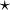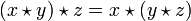# Associativity

(Redirected from Associative)

In algebra, associativity is a property of binary operations. If$\star$ is a binary operation then the associative property is the condition that$(x \star y) \star z = x \star (y \star z) \,$

for all x, y and z.

Examples of associative operations are addition and multiplication of integers, rational numbers, real and complex numbers. In this context associativity is often referred to as the associative law. Function composition is associative.

An important example of an algebraic structure in which the multiplication is not associative is the octonions.

## Related properties

An operation is left alternative if$(x \star x) \star y = x \star (x \star y) \,$

for all x and y: it is right alternative if$(y \star x) \star x = y \star (x \star x) . \,$

An operation is power-associative if$(x \star x) \star x = x \star (x \star x) \,$

for all x. In such cases the expression xn is well-defined for all positive integers n.

## Operator associativity

When an operation is not associative a convention is required to disambiguate an expression such as x * y * z, and this convention may be described as the associativity of the operator "*". Left-to-right associativity means that the expression is to be interpreted as (x * y) * z (which is the normal arithmetical convention for subtraction and division) and right-to-left associativity means x * (y * z) (which is the normal arithmetical convention for exponentiation). Such conventions may be important in computer programming languages where a mathematically associative operator may have a non-associative numerical implementation.